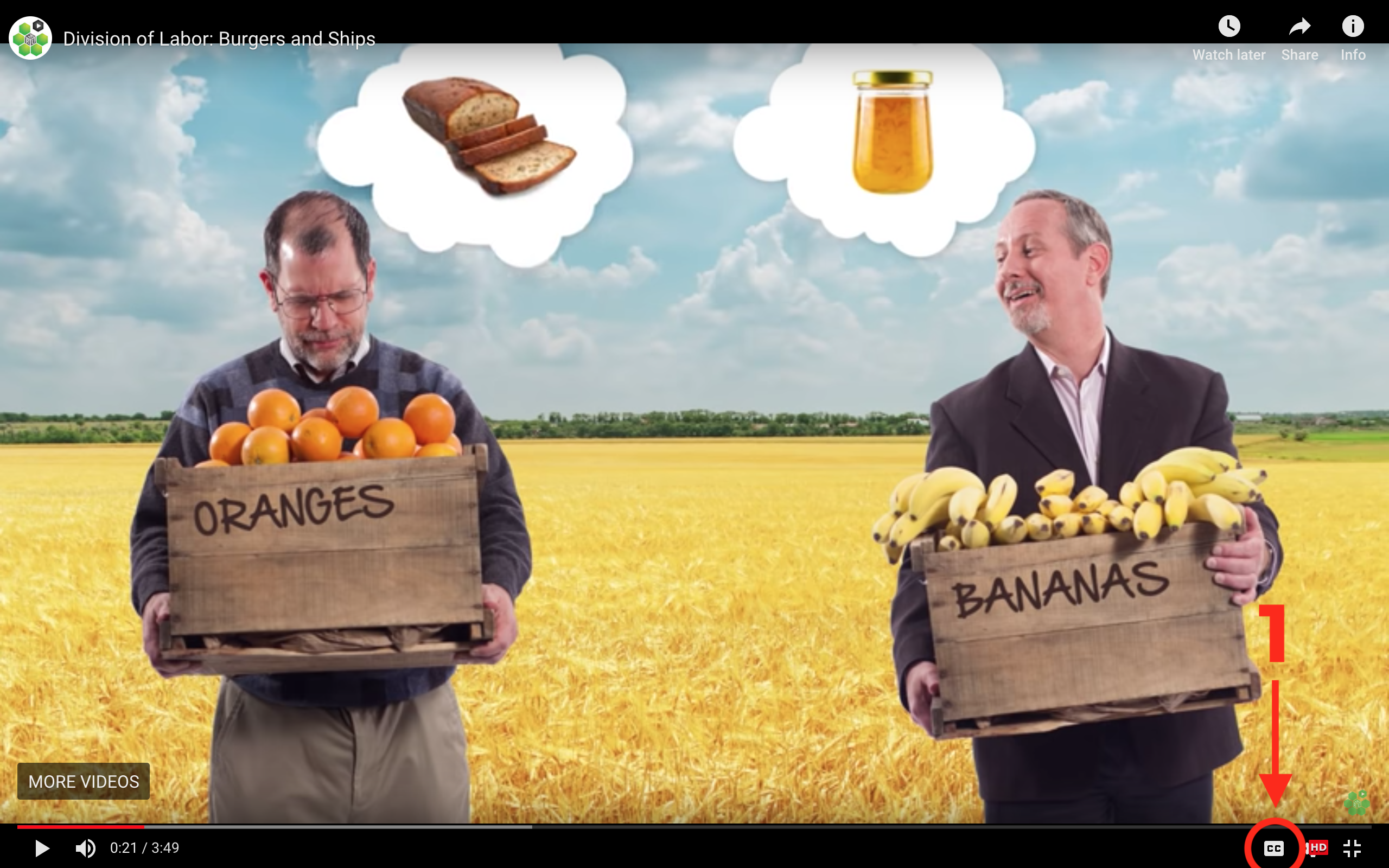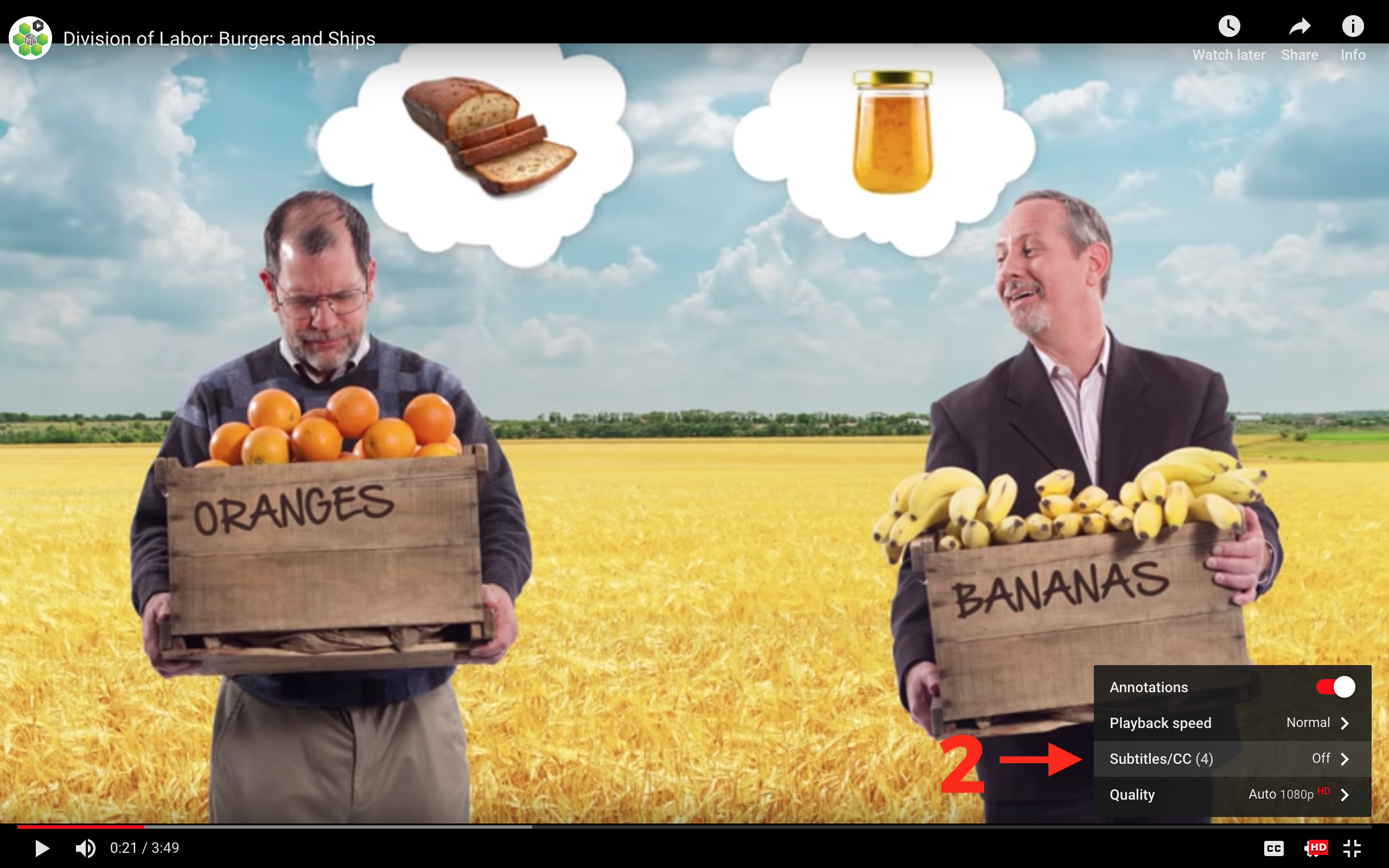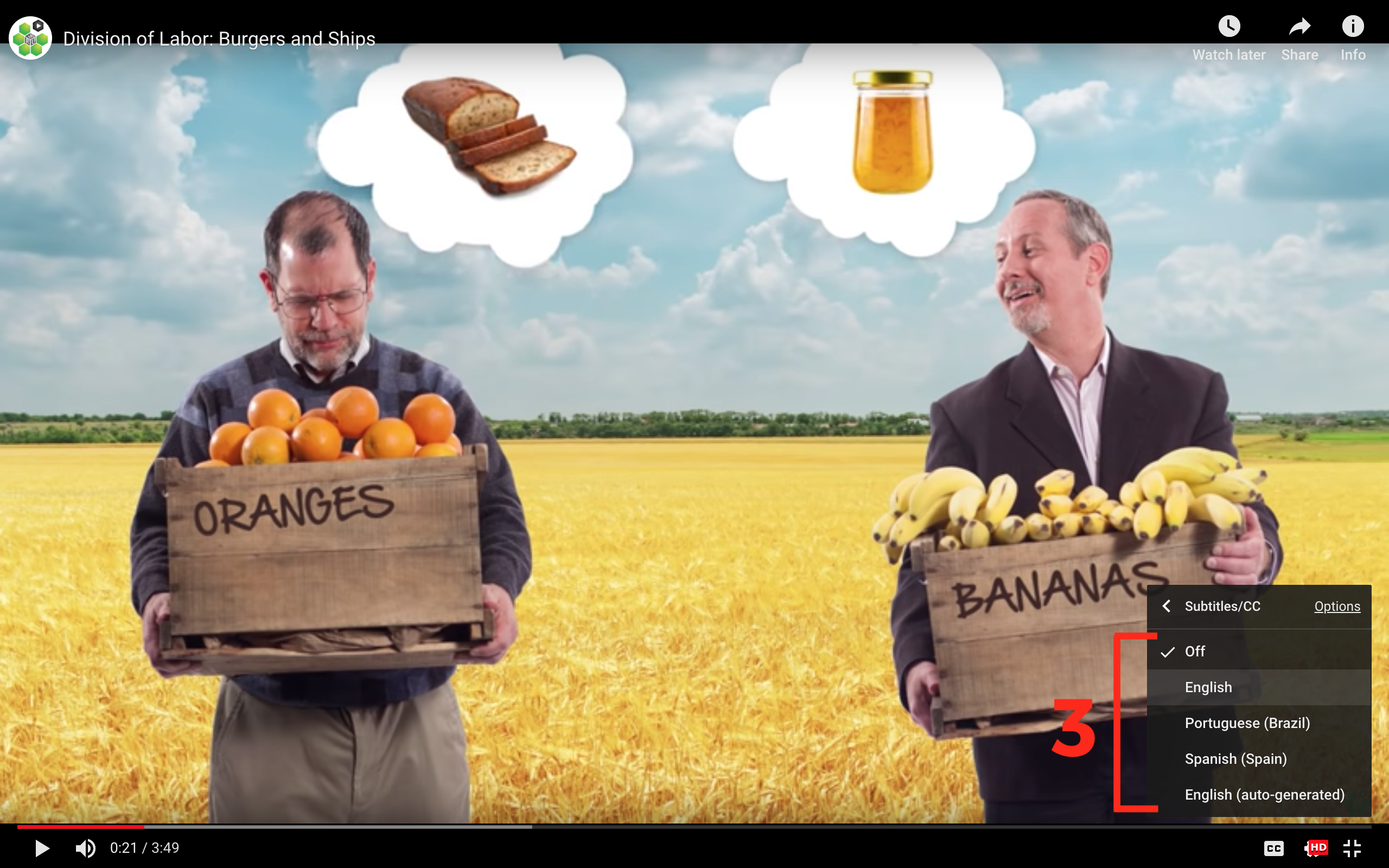## Profit Maximization

Profit Maximization: The process by which firms determine the price and output quantity that will yield the highest possible profit. This is done by setting Marginal Revenue equal to Marginal Cost. This is from the video “Maximizing Profit Under Competition” in the Principles of Microeconomics course.

## Transcript

We learned last time that a firm in a competitive market doesn't have much control over it's price. It must accept the market price. So its decision about profit maximization turns into a decision about what quantity to choose, and that's what we're going to be focusing on now.

So what is profit? Profit is total revenue minus total cost. Total revenue is just price times the quantity sold. Total cost has two parts. First are the fixed costs. These are costs that do not vary with output. So, for example, suppose you are the owner of this small oil well and you have to pay rent for the land on which the oil well sits. Those rental costs -- you have to pay them regardless of how much the oil well is producing. Every month you have to pay some rental cost whether you're producing one barrel of oil per month, 10 barrels of oil per month, 11 barrels of oil per month. It doesn't matter. You still have to pay the same rental cost. Indeed, even if you don't produce any oil that month, if your oil well breaks down, you still have to pay those rental costs.

So the rental costs are fixed costs. They don't vary with the quantity produced. By the way, notice that even if you owned the land, if you could have rented it to someone else, then that would be an opportunity cost. So your calculation of profit should also include opportunity costs. That's what makes the economic calculation of profit, by the way, differ from the accounting definition of profit. The economic notion of profit includes opportunity costs.

Okay, what else? Well, variable costs -- these are the cost that do vary with output. So for example, the electricity cost for pumping oil -- the more oil you pump, the faster you get your rig to go, the more electricity you're going to use up. If you run it 24 hours a day, you're going to use more electricity than if you only run the pump 12 hours a day. Transportation cost -- you got to go and get the oil, truck it out of there, move it and so forth. So these costs are all costs which vary with output, which typically will increase the more output that you produce. Those are your variable costs. So just to summarize on cost, total cost is equal to your fixed costs plus your variable costs and these depend upon output.

Okay, so how do we maximize profit? Well, we're not going to use calculus in this class, but for those of you who do know calculus, I want to do a quick aside -- show you actually how useful calculus is and show you an easy way of answering this problem. So we know the profit is total revenue minus total cost and both of these are functions of the quantity produced. Now in calculus how do we maximize a function? Think back to your calculus class. You take the derivative of that function and you set it equal to zero. So in this case, we want to take the derivative of profit with respect to quantity and set that equal to zero. So derivative of profit with respect to quantity -- that's just the derivative of total revenue with respect to quantity minus the derivative of total cost with respect to quantity.

Now in economics, we have special names for these two derivatives. The derivative of total revenue with respect to quantity is simply called marginal revenue. And the derivative of total cost with respect to quantity is called marginal cost. So we want to find the quantity such that marginal revenue minus marginal cost is zero, or in other words, we want to find the quantity such that marginal revenue is equal to marginal cost. In other words, the quantity, which maximizes profit, is the one where marginal revenue is equal to marginal cost.

Now I'm about to give you a more intuitive explanation, especially for those of you who don't get no calculus, but for those of you who do, this is just exactly what you were to do in calculus -- you take the derivative, set it equal to zero. Okay let's get to more intuition. When the firm produces an additional unit of output, there are additional revenues and additional costs. Profit maximization is all about comparing these additional revenues and costs, and we have names for these. Marginal revenue is the addition to total revenue from selling an additional unit of output. Marginal cost is the addition to total cost from producing an additional unit of output. Profits are maximized at the level of output where marginal revenue is equal to marginal cost. Now why is this? Well, let's suppose that marginal revenue is not equal to marginal cost and let’s show that you can't be profit maximizing if that's the case.

For example, if marginal revenue is bigger than marginal cost, you're not profit maximizing -- producing more will add to your profit. Why? Well, remember marginal revenue is the addition to revenue from producing another unit. Marginal cost is the addition to cost from producing another unit. If marginal revenue is bigger than marginal cost, that says producing that unit adds more to your revenues than it does to your costs. In other words, you could increase profit by producing more. So if marginal revenue is ever bigger than marginal cost, you want to produce more. On the other hand, suppose marginal revenue is less than marginal cost, or to put it the other way, suppose marginal cost is bigger than marginal revenue. Well then, you're not profit maximizing because producing less will add to your profit.

Why is this? Well, think about marginal cost. If you were to produce one unit less your costs would fall by marginal cost, your revenues would also fall by marginal revenue, but since marginal cost is bigger than marginal revenue, your costs by producing one unit less fall by more than your revenues fall. So if your costs are going down by more than your revenues are going down, you're again increasing profit. So if marginal revenue is ever less than marginal cost, you want to produce less -- you'll be increasing your profit by producing less. So, if marginal revenue is bigger than marginal cost, you're not profit maximizing. If marginal revenue is less than marginal cost you're not profit maximizing. You can only profit maximize if marginal revenue is equal to marginal cost.

Now let's put all this in a diagram beginning with marginal revenue. Now for a competitive firm, this is going to be easy because remember, that a competitive firm is small relative to the total market. That means it can double its production easily and not push down the market price. As a result, for a competitive firm, marginal revenue is equal to the market price.

So for example, suppose the firm is producing two units of output and it decides to produce a third unit, what's the additional revenue from that third unit? It's the price. It's the price it gets for that barrel of oil. What about if it produces a fourth barrel of oil? What does it get? What's the addition to revenue? It's the price of a barrel of oil. What about the fifth unit? Again, the price is the addition to revenue, is marginal revenue. So, marginal revenue for a competitive firm is equal to the price and it's flat -- it doesn't change when the firm changes its output because the firm is small relative to the market.

Now what about marginal cost? Well, a typical shape of a marginal cost curve would be upward sloping like this. Again, think about our stripper oil well. We can produce more from that oil well, but there's a limit. We can only run it so quickly. We have to push it really hard when we start to produce more. So we can easily produce, you know, three, or four units, but in order to produce six, seven, eight, or nine barrels of oil from that oil well, we're going to have to run it really quickly, we're going to have to put in a lot of electricity, we're going to have to do a lot of maintenance and so forth. So our costs will tend to increase. We can't produce an unlimited amount of oil at the same cost from this oil well. Our costs are going to go up, are going to rise, our additional costs are going to rise the more we want to produce from that oil well. So this is a typical shape of a marginal cost curve.

Now, where's profit maximization? Well, profit is maximized where marginal revenue is equal to marginal cost. In this case, for a competitive firm, marginal revenue is equal to price. So profit is maximized where price is equal to marginal cost or at this point right here. Now let's think about that intuitively. On the left hand side, this is the additional revenues from selling a barrel of oil. These are the additional costs from selling a barrel of oil. So you want to compare -- revenues bigger than costs, therefore sell more. Revenues bigger than costs, therefore sell more. Revenues bigger than costs. You keep selling more until you reach this point.

Do you want to go further? No. Here, costs are bigger than revenues. So by selling less, you can reduce your costs by more than you'd reduce your revenues and therefore profit goes up going this way and that's why this point, where marginal revenue is equal to marginal cost, or price is equal to marginal cost, that's the point where profit is maximized.

Now remember way back in the first talk, we wanted to explain a firm’s behavior. So let's look how maximizing profit explains the firm’s behavior. Suppose the market price is \$50 per barrel. Well, then in order to maximize profit, the firm chooses the quantity -- in this case, about eight barrels of oil -- such that marginal revenue is equal to marginal cost, bearing in mind that for the competitive firm, marginal revenue is equal to price. So to profit maximize the firm produces a quantity of about eight barrels of oil. Now suppose that the market price goes up to \$100. Now in order to profit maximize, the firm increases its production along its marginal cost curve keeping this relationship the same so price is still equal to marginal cost. Price has gone up to 100, but because the firm has expanded along its marginal cost curve, marginal cost has gone up as well.

So this again is the profit maximizing point when the price is equal to 100. When the price is equal to 100, the profit maximizing quantity is just under 10 barrels of oil. So profit maximization explains what the firm does when the price, when the market price, changes. We now know how to find the profit maximizing quantity -- look for the quantity where marginal revenue is equal to marginal cost, which is the same for the competitive firm where price is equal to marginal cost.

Now we want to ask, what is the size of the profit? This raises a subtle point. You can be maximizing profits and actually have a loss. That is, the best that you can do might be a loss. So we want to show on the diagram how large your profits or how large your losses are when you are maximizing profits. In order to do that, we need to introduce another concept and another curve -- average cost. Average cost is simply the cost per unit of output. That is the total cost divided by Q, the quantity of the output. So average cost again -- total cost divided by Q. Adding the average cost curve to our graph will let us show profit on the graph. And that's what we want to do, and that's what we'll do in the next talk. Thanks.

## Subtitles

### Turn captions on or off:

1. If captions are available the (CC) icon will be visible on the player.
2. To turn captions on, tap (CC).
3. To turn captions off, tap (CC) again.### Select caption language:

1. Click the settings icon (⚙) at the bottom of the video screen.
2. Click Subtitles/CC.
3. Select a language.## Contribute Translations!

Join the team and help us provide world-class economics education to everyone, everywhere for free! You can also reach out to us at support@mru.org for more info.

## Accessibility

We aim to make our content accessible to users around the world with varying needs and circumstances.

Currently we provide:

Are we missing something? Please let us know at support@mru.org